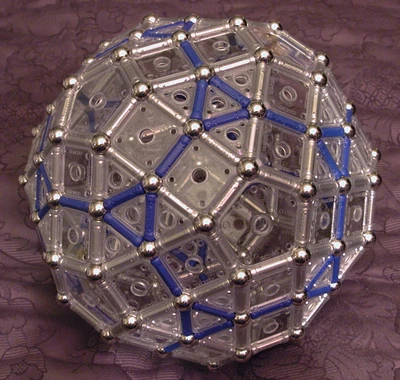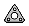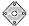## Augmented Truncated Dodecahedron -- Discussion

There are two (regular) ways of augmenting a Truncated Dodecahedron with pentagonal cupolae: cupola pairs connected square-to-square (CS2S) and connected triangle-to-triangle (CT2T).

The Truncated Dodecahedron may also be augmented with pentagonal rotundae in two (regular) ways: rotunda pairs connected pentagon-to-pentagon (RP2P) and connected triangle-to-triangle (RT2T), producing polyhedra that are far from spherical. These models do not appear in this entry, because I haven't tried to build them myself (yet).

## Augmented Truncated Dodecahedron (Cupolae Square-to-Square)

 Augmented Truncated Dodecahedron (Cupolae Square-to-Square)Truncated Dodecahedron augmented with outward Pentagonal Cupolae, aligned Square-to-Square Type Polyhedron Panels 80 ×60 ×12 ×Rods 270 ×Spheres 120 ×Author PolyClare 03:06, 20 January 2009 (UTC)

To the right is a Truncated Dodecahedron augmented with Pentagonal Cupolae. In this case, all the pentagons are aligned so that a sqaure from one cupola is connected to a square from its neighboring cupola.

It is interesting to note that in this model, pairs of connected squares are coplanar, forming rectangles of size 1×2 units. Additionally, blocks of four trangles are also coplanar, forming equilateral triangles of edge length 2 units. The overall result is that this model may be viewed as a stretched (no longer regular) Rhombicosidodecahedron, shown for ease of comparison below:

Note that augmenting the Truncated Dodecahedron with even one pentagonal cupola aligned square-to-dodecahedron ends up with cupola triangles that are coplanar with the Truncated Dodecahedron triangles. Therefore there are no Truncated Dodecahedra augmented with pentagonal cupolae oriented this way among the 92 Johnson Solids.

## Augmented Truncated Dodecahedron (Cupolae Triangle-to-Triangle)

 Augmented Truncated Dodecahedron (Cupolae Triangle-to-Triangle)Truncated Dodecahedron augmented with outward Pentagonal Cupolae, aligned Square-to-Square Type Polyhedron Panels 80 ×60 ×12 ×Rods 270 ×Spheres 120 ×Author PolyClare 03:06, 20 January 2009 (UTC)

To the right is a Truncated Dodecahedron augmented with Pentagonal Cupolae. In this case, all the pentagons are aligned so that a triangle from one cupola is connected to a square from its neighboring cupola.

It is interesting to note that this model is nonconvex, since pairs of triangles from connected cupolae are NOT coplanar. However, these triangle pairs are very close to being coplanar (see further discussion and photos below). The overall result is that using Geomag one may carefully replace pairs of triangles with Rhombic Panels, resulting in an intriguing near miss described in the entry entitled Pseudosphere From Geomag Box.

Note that augmenting the basic Truncated Dodecahedron with just one pentagonal cupola, aligned triangle-to-dodecahedron ends up with a convex polyhedron. It is the triangle-to-triangle pairs of multiple adjacent pentagonal cupolae that make the Augmented Truncated Dodecahedron CS2S nonconvex. This is why there are only four Truncated Dodecahedron-based polyhedra listed among the 92 Johnson Solids: J68 "augmented truncated dodecahedron" (one cupola), J69 "parabiaugmented truncated dodecahedron" (two cupolae, opposite ends), J70 "metabiaugmented truncated dodecahedron" (two nonadjacent cupolae, separated by one decagon), and J71 "triaugmented truncated dodecahedron" (three nonadjacent cupolae). These are the four ways to attach pentagonal cupolae to a Truncated Dodecahedron without any pentagonal cupola being adjacent to another.

## Model Gallery and Discussion

First, for comparison purposes, displayed below is the Truncated Dodecahedron which has been "diminshed" by pentagonal Cupolae aligned Square-to-Square (CS2S):

 Truncated Dodecahedron Diminished by Pentagonal Cupolae, CS2SLeft viewRight View

### Augmented Truncated Dodecahedron (Cupolae Square-to-Square)

Below are two wall-eyed stereo pairs of the Augmented Truncated Dodecahedron (CS2S). You can see that this model is the same as the previous one above, except that the pentagonal cupolae point outward instead of inward (i.e. "augmented by cupolae" instead of "diminished by cupolae").

 Truncated Dodecahedron Augmented by Pentagonal Cupolae, CS2S (View 1)Left viewRight View

 Truncated Dodecahedron Augmented by Pentagonal Cupolae, CS2S (View 2)Left viewRight View

### Augmented Truncated Dodecahedron (Cupolae Triangle-to-Triangle)

If you detach the pentagonal cupolae from the previous CS2S model above, and twist and reattach each cupola so that they connect at corresponding triangles (instead of at squares), you get the Augmented Truncated Dodecahedron (Cupolae Triangle-to-Triangle) model. Below is a photo of a model with JUST ONE cupola twisted:

 One Twisted Pentagonal CupolaBelow are two wall-eyed stereo pairs of the Augmented Truncated Dodecahedron (Cupolae Triangle-to-Triangle).

 Truncated Dodecahedron Augmented by Pentagonal Cupolae, CT2T (View 1)Left viewRight View

 Truncated Dodecahedron Augmented by Pentagonal Cupolae, CT2T (View 2)Left viewRight View

### Noncoplanarity of Triangle Pairs in the CT2T Model

If you look very close and carefully at the preceding photos of the Cupolae-Triangle-to-Triangle model, you may suspect that the triangle pairs are noncoplanar, resulting in a nonconvex model. See closeup below:

 Noncoplanar T2T Triangles: CLOSEUPExtracting just the corner triangle from the Truncated Dodecahedron, along with its 9 surrounding polygons (3 adjacent squares attached along one edge and 3 pairs of triangles attached at a vertex), you can see that this figure can be flattened out to a planar form:

If any of the squares are flexed at even a slight angle to the central triangle, the outer triangle pairs will be forced to buckle, producing a figure that cannot be convex. Therefore the Triangle-to-Triangle form of the Augmented Truncated Dodecahedron is nonconvex. This is why the Pseudosphere From Geomag Box is a near miss instead of a working convex model.